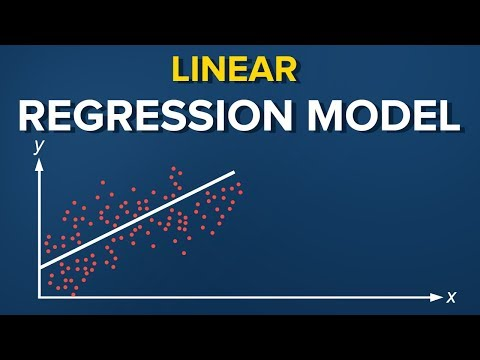# The simple linear regression model

Let’s start with some dry theory. A linear regression model is a linear approximation of a causal relationship between two or more variables. Regressions models are highly valuable, as they are one of the most common ways to make inferences and predictions.

The process goes like this. You get sample data, come up with a model that explains the data, and then make predictions for the whole population based on the model you’ve developed.

There is a dependent variable, labeled Y, being predicted, and independent variables, labeled x1, x2, and so forth. These are the predictors. Y is a function of the X variables, and the regression model is a linear approximation of this function.

The easiest regression model is the simple linear regression: Y is equal to beta zero plus beta one times x plus epsilon.

Let’s see what these values mean. Y is the variable we are trying to predict and is called the dependent variable. X is an independent variable. When using regression analysis, we want to predict the value of Y, provided we have the value of X.

### But to have a regression, Y must depend on X in some causal way.

Whenever there is a change in X, such change must translate into a change in Y.

Think about the following equation: the income a person receives depends on the number of years of education that person has received. The dependent variable is income, while the independent variable is years of education. There is a causal relationship between the two. The more education you get, the higher income you are likely to receive. This relationship is so trivial that it is probably the reason you are watching this course, right now. You want to get a higher income, so you are increasing your education.

Now, let’s pause for a second and think about the reverse relationship. What if education depends on income. This would mean the higher your income, the more years you spend educating yourself. Putting high tuition fees aside, wealthier individuals don’t spend more years in school. And, high school and college take the same number of years, no matter your tax bracket. Therefore, a causal relationship like this one is faulty, if not plain wrong. Hence, it is unfit for regression analysis.

Let’s go back to the original example. Income is a function of education. The more years you study, the higher income you will receive. This sounds about right.

### Alright.

What we haven’t mentioned, so far, is that, in our model, there are coefficients. Beta one is the coefficient that stands before the independent variable. It quantifies the effect of education on income. If beta one is 50, then for each additional year of education, your income would grow by \$50. In the USA, the number is much bigger, somewhere around 3 to 5,000 dollars. So, for each additional year you spend on education, your yearly income is expected to rise by three to five thousand dollars. And that’s not considering higher education or tailored courses, like this one.

The other two other components are the constant beta zero and the error – epsilon.

In this example, you can think of the constant beta zero as the minimum wage. No matter your education, if you have a job, you will get the minimum wage. This is a guaranteed amount.

So, if you never went to school and plug an education value of 0 years in the formula, the regression will predict that your income will be the minimum wage. Makes sense, right?

The last term is the epsilon. This represents the error of estimation. The error is the actual difference between the observed income and the income the regression predicted. On average, across all observations, the error is 0. If you earn more than what the regression has predicted, then someone earns less than what the regression predicted. Everything evens out.

### Alright.

The original formula was written with Greek letters. What does this tell us? It was the population formula. But we know statistics is all about sample data. In practice, we use the linear regression equation.

It is simply y hat equals b zero plus b one times x.

You heard right. The y here is referred to as y hat. Whenever we have a hat symbol, it is an estimated or a predicted value.

b zero is the estimate of the regression constant beta zero, while b one is the estimate of beta one, and x is the sample data for the independent variable.

You now know what simple linear regression is. Curious to learn more? Check out our all-in-one Data Science Training.#### Data Science PR

This site uses Akismet to reduce spam. Learn how your comment data is processed.

#### Recent posts

###### Hedera Hashgraph Price Analysis 2021 – HBAR Token Price Forecast 2021 -Hbar Price Prediction 2021Don't be shy, get in touch. We love meeting interesting people and making new friends.